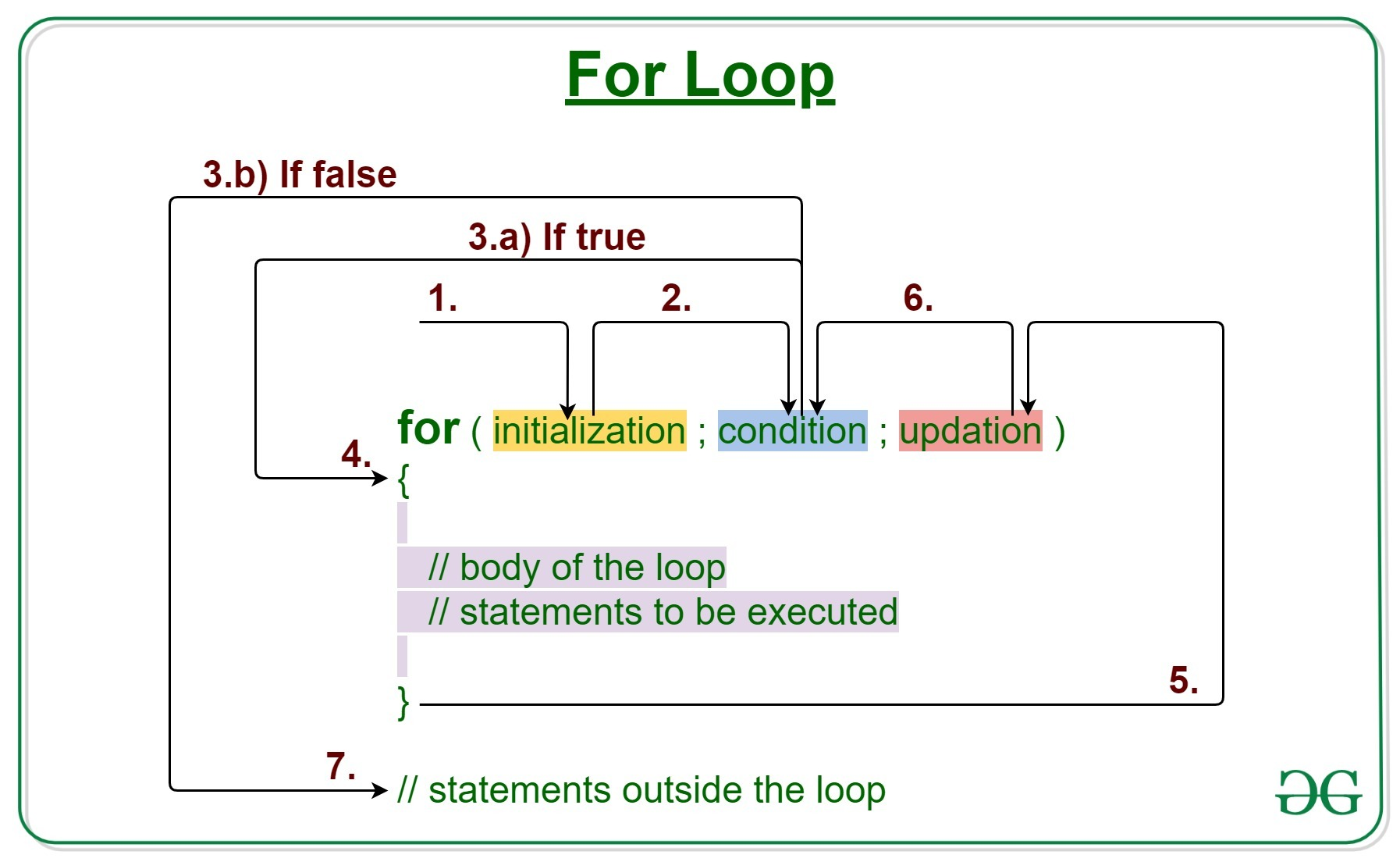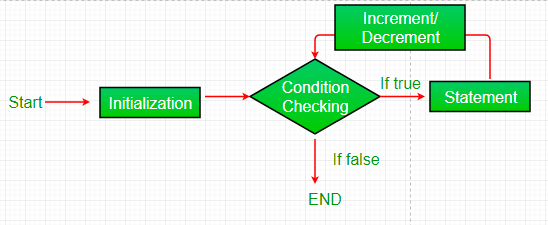Open in App
Not now

# Java For loop with Examples

• Difficulty Level : Easy
• Last Updated : 06 Jun, 2022

Loops in Java come into use when we need to repeatedly execute a block of statements. Java for loop provides a concise way of writing the loop structure. The for statement consumes the initialization, condition and increment/decrement in one line thereby providing a shorter, easy to debug structure of looping.Syntax:

```for (initialization expr; test expr; update exp)
{
// body of the loop
// statements we want to execute
}```

The various parts of the For loop are:

1. Initialization Expression: In this expression, we have to initialize the loop counter to some value.

Example:

`int i=1;`

2. Test Expression: In this expression, we have to test the condition. If the condition evaluates to true then, we will execute the body of the loop and go to update expression. Otherwise, we will exit from the for loop.

Example:

`i <= 10`

3. Update Expression: After executing the loop body, this expression increments/decrements the loop variable by some value.

Example:

`i++;`

How does a For loop execute?

1. Control falls into the for loop. Initialization is done
2. The flow jumps to Condition
3. Condition is tested.
1. If Condition yields true, the flow goes into the Body
2. If Condition yields false, the flow goes outside the loop
4. The statements inside the body of the loop get executed.
5. The flow goes to the Updation
6. Updation takes place and the flow goes to Step 3 again
7. The for loop has ended and the flow has gone outside.

Flow chart for loop (For Control Flow):Example 1:This program will print 1 to 10

## Java

 `/*package whatever //do not write package name here */` `// Java program to write a code in for loop from 1 to 10`   `class` `GFG {` `    ``public` `static` `void` `main(String[] args)` `    ``{` `        ``for` `(``int` `i = ``1``; i <= ``10``; i++) {` `            ``System.out.println(i);` `        ``}` `    ``}` `}`

Output

```1
2
3
4
5
6
7
8
9
10```

Example 2: This program will try to print “Hello World” 5 times.

## Java

 `// Java program to illustrate for loop` `class` `forLoopDemo {` `    ``public` `static` `void` `main(String args[])` `    ``{` `        ``// Writing a for loop` `        ``// to print Hello World 5 times` `        ``for` `(``int` `i = ``1``; i <= ``5``; i++)` `            ``System.out.println(``"Hello World"``);` `    ``}` `}`

Output

```Hello World
Hello World
Hello World
Hello World
Hello World```

Time Complexity: O(1), Auxiliary Space : O(1)

Dry-Running Example 1: The program will execute in the following manner.

```1. Program starts.
2. i is initialized with value 1.
3. Condition is checked. 1 <= 5 yields true.
3.a) "Hello World" gets printed 1st time.
3.b) Updation is done. Now i = 2.
4. Condition is checked. 2 <= 5 yields true.
4.a) "Hello World" gets printed 2nd time.
4.b) Updation is done. Now i = 3.
5. Condition is checked. 3 <= 5 yields true.
5.a) "Hello World" gets printed 3rd time
5.b) Updation is done. Now i = 4.
6. Condition is checked. 4 <= 5 yields true.
6.a) "Hello World" gets printed 4th time
6.b) Updation is done. Now i = 5.
7. Condition is checked. 5 <= 5 yields true.
7.a) "Hello World" gets printed 5th time
7.b) Updation is done. Now i = 6.
8. Condition is checked. 6 <= 5 yields false.
9. Flow goes outside the loop. Program terminates.```

Example 3: The following program prints the sum of x ranging from 1 to 20.

## Java

 `// Java program to illustrate for loop.` `class` `forLoopDemo {` `    ``public` `static` `void` `main(String args[])` `    ``{` `        ``int` `sum = ``0``;`   `        ``// for loop begins` `        ``// and runs till x <= 20` `        ``for` `(``int` `x = ``1``; x <= ``20``; x++) {` `            ``sum = sum + x;` `        ``}` `        ``System.out.println(``"Sum: "` `+ sum);` `    ``}` `}`

Output

`Sum: 210`

Enhanced For Loop or Java For-Each loop

Java also includes another version of for loop introduced in Java 5. Enhanced for loop provides a simpler way to iterate through the elements of a collection or array. It is inflexible and should be used only when there is a need to iterate through the elements in a sequential manner without knowing the index of the currently processed element.

Note: The object/variable is immutable when enhanced for loop is used i.e it ensures that the values in the array can not be modified, so it can be said as a read-only loop where you can’t update the values as opposed to other loops where values can be modified.

Syntax:

```for (T element:Collection obj/array)
{
// loop body
// statement(s)
}```

Let’s take an example to demonstrate how enhanced for loop can be used to simplify the work. Suppose there is an array of names and we want to print all the names in that array. Let’s see the difference between these two examples by this simple implementation:

## Java

 `// Java program to illustrate enhanced for loop`   `public` `class` `enhancedforloop {`   `    ``public` `static` `void` `main(String args[])` `    ``{` `        ``String array[] = { ``"Ron"``, ``"Harry"``, ``"Hermoine"` `};`   `        ``// enhanced for loop` `        ``for` `(String x : array) {` `            ``System.out.println(x);` `        ``}`   `        ``/* for loop for same function` `        ``for (int i = 0; i < array.length; i++)` `        ``{` `            ``System.out.println(array[i]);` `        ``}` `        ``*/` `    ``}` `}`

Output

```Ron
Harry
Hermoine```

Time Complexity: O(1), Auxiliary Space : O(1)

Recommendation: Use this form of statement instead of the general form whenever possible. (as per JAVA doc.)

JAVA Infinite for loop:

This is an infinite loop as the condition would never return false. The initialization step is setting up the value of variable i to 1, since we are incrementing the value of i, it would always be greater than 1 so it would never return false. This would eventually lead to the infinite loop condition.

Example:

## Java

 `// Java infinite loop` `class` `GFG {` `    ``public` `static` `void` `main(String args[])` `    ``{` `        ``for` `(``int` `i = ``1``; i >= ``1``; i++) {` `            ``System.out.println(``"Infinite Loop "` `+ i);` `        ``}` `    ``}` `}`

There is another method for calling the Infinite for loop

If you use two semicolons ;; in the for loop, it will be infinitive for loop.

Syntax:

```for(;;){
//code to be executed
}  ```

Example:

## Java

 `public` `class` `GFG {`   `    ``public` `static` `void` `main(String[] args)` `    ``{` `        ``for` `(;;) {` `            ``System.out.println(``"infinitive loop"``);` `        ``}` `    ``}` `}`

https://youtu.be/JkSQ8KtOA14

My Personal Notes arrow_drop_up
Related Articles